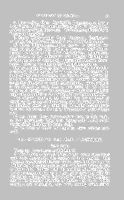# University of Virginia Library

### PROF. VENABLE.

This School embraces two distinct departments or courses:

• 1. Pure Mathematics.

• 2. Mixed Mathematics.

I. Pure. Mathematics.—In the course of Pure Mathematics
there are three classes, Junior, Intermediate and
Senior.

In the Junior Class are studied the Theory of Arithmetical
Operations and Notation, Algebra, Geometry and
Geometrical Conic Sections, with discussions of the Ancient
Geometrical Analysis, the Theory of Transversals, and other
subjects of Modern Geometry. The preparation desirable
for this class is the thorough study of Arithmetic, of Algebra
through Equations of the Second Degree, and of the first
four books of Legendre or Euclid.

In the Intermediate Class the studies are Plane and
Spherical Trigonometry with Applications, Analytical Geometry
of two dimensions, the Theory of Equations and
Elements of Descriptive Geometry.

In the Senior Class the subjects of study are Analytical
Geometry of three Dimensions, the Differential and Integral
Calculus with applications to the Theory of Probabilities,
and the Determination of Mean Values and Centres of
Gravity, and the Calculus of Variations. Lectures are given
to this class on the History of Mathematics, and on some
points in Controversial Mathematics, with brief discussions
of the elements of Trilinear Coördinates, of the Theory of
Determinants, the Theory of Complex Functions, and of the
principles of the Method of Quaternions.

Text-Books in Pure Mathematics:

1. Junior Class—Venable's Higher Arithmetic, Todhunter's
Algebra, Legendre's Geometry. For reference and
examples, Pott's Euclid, Taylor's Conic Sections.

352. Intermediate Class—Snowball's Trigonometry, Law's
Logarithms, Puckle's Conic Sections (Analytical Geometry),
Church's Descriptive Geometry. For reference, Todhunter's
Theory of Equations.

3. Senior Class—Aldis' Solid Geometry, Courtenay's
Calculus, Todhunter's Differential and Integral Calculus.

II. Mixed Mathematics.—This course is designed for
those students who may desire to prosecute their studies
beyond the limits of the Pure Mathematics. It embraces
Applications of the Differential and Integral Calculus to
selected portions of Mechanics, Physics and Physical Astronomy.
There is one class in the Mixed Mathematics.

The instruction in each class in the School of Mathematics
is conveyed partly by lectures and partly by the systematic
study of approved text-books, the student being assisted by
full and frequent explanations from the Professor, and being
constantly subjected to rigid examinations. The progress
of the student in each class is also tested by his being
required to perform written exercises, in which the principles
acquired are applied to the solution of particular problems.

Any student entering the school has the privilege of
attending all or any of the classes, and if prepared to enter